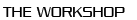﻿ 11th Workshop on Numerical Methods for Evolution Equations ::Past Workshops

Privacy noticeList of Talks

• Georgios Akrivis
The linearly implicit two-step BDF method for harmonic maps into spheres.
On the Nitsche’s approach for a finite element scheme for Vlasov-Maxwell system.
• Lehel Banjai
Runge-Kutta convolution quadrature for hyperbolic problems based on Gauss methods.
• Konstantinos Chrysafinos
Fully discrete approximations of the velocity tracking problem with pointwise-integral control constraints in time-space.
• Alexandre Ern
Invariant-domain preserving Runge-Kutta methods.
• Emmanuil Georgoulis
Hypocoercivity-exploiting stabilised finite element methods for Kolmogorov equation.
• Patrick Joly
Remarks on explicit time stepping for the DG space discretisation of linear Friedrichs systems.
• Theodoros Katsaounis
Optimisation methods for elastodynamics.
• David Ketcheson
How to approximate any evolution PDE by a hyperbolic PDE.
• Christian Kreuzer
Inf-sup theory for the Biot equations: analysis and discretisation.
• Ulrich Langer
Space-time finite element solvers for parabolic optimal control problems.
• Mats Larson
Strong stabilization of cut elements and explicit time stepping.
A priori and a posteriori error estimates for discontinuous Galerkin time-discrete methods via maximal regularity.
• María López-Fernández
Generalized convolution quadrature for sectorial problems.
• Christian Lubich
Time-dependent electromagnetic scattering from thin layers.
• Charalambοs Makridakis
Neural network approximations to evolution problems.
• Vanja Nikolić
Asymptotic-preserving finite element discretization of quasilinear acoustic equations.
• Alexander Ostermann
An exponential integrator/WENO discretization for dispersive sonic-boom simulation.
• Daniel Peterseim
Computational polyconvexification of isotropic functions.
• Tristan Pryer
Structure-preservation in non-Newtonian flows.
• Mariya Ptashnyk
Numerical analysis of a time-stepping method for the Westervelt equation with time-fractional damping.
• Thomas Wihler
A discrete calculus for variable-order dG time stepping methods.
• Georgios Zouraris
On the convergence of a linearly implicit finite element method approximating a nonlinear Schrödinger equation.